Breaking News
Home / Civil Engineering / Bar Bending Schedule / Bar Bending Schedule B.B.S

# Bar Bending Schedule B.B.S

### Introduction to B.B.S

B.B.S The word B.B.S Plays a significant role in any Constructions of the High rise buildings. B.B.S refers to B.B.S. Well, What is the use of B.B.S ? Why we use B.B.S ? What is B.B.S ?

In this article we are majorly focused on how & where to start & what are the basics.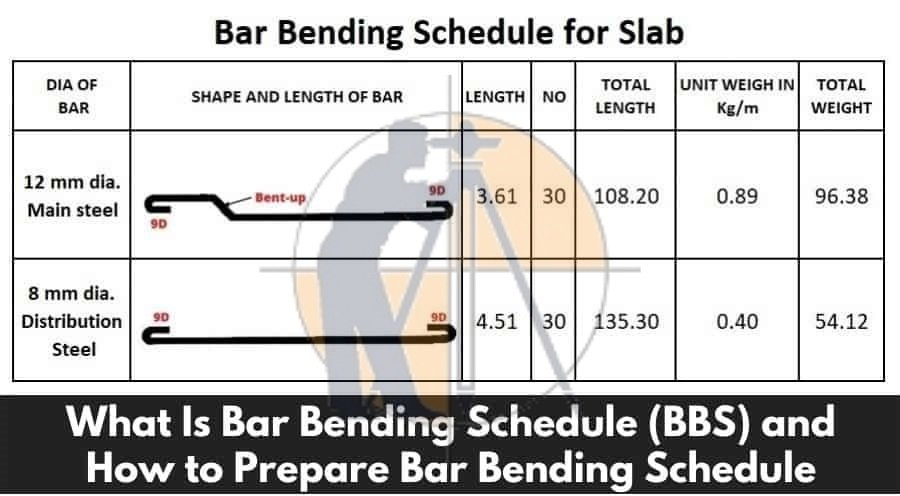It’s a lengthy article, so brace yourself for at least 10 minutes. Let’s Get started.

First of all, Bar is any type of rebar which is used as a reinforcement in R.C.C. The bar may be a Mild Steel bar or H.Y.S.D bar or T.M.T Bar.

B.B.S is termed as ( Calculations of the total Steel required for the construction of a building )  We use steel to make concrete to be reinforced & for tension requirements. But how much steel required for constructing 15 floors building ? How much Steel I have to order ? All these questions are answered in B.B.S

### Thumb Rules For Civil Engineers In Building Construction

In B.B.S, the bars are organized for each structural units ( Beams or columns or slabs or footings etc.) & detailed list is prepared which specifies the Bar location ( Bar in footings, slabs, beams or columns ), Bar Marking ( to identify the bar in accordance with the drawing ), Bar Size ( length of the bar used ), Quantity ( No. of Bars used ), Cutting length, Type of Bend & Shape of the bar in reinforcement drawings.

### How B.B.S Changed from 1950 – 2022

From 1950 to 2022 lots of modifications and enhancements happening in our world. In 1950, three-storiey buildings are high rise buildings now we are constructing a building with 200 floors+. There is a massive growth in the construction industry. Due to the vast increase in world population demands increased facilities, more need for space & more constructions.

Father of Estimation B.N. Data has given certain recommendations for the usage of steel in different components of the buildings. But, he didn’t mention any values if we use more bars in a single structural member.

#### 0.8% of total volume of concrete

At that time we’ve used only four bars in columns; now we are using 12+ bars in the columns based on load analysis. So, the percentage of the steel is increased in a column which reveals that the above-cited values are outdated. ( They are outdated “not wrong”) He wrote that book in 1950. Now we are in 2022. He gave recommendations according to the potentiality of the construction at that time. Now we are constructing 200 + floors in the small area.

# Bar Bending Schedule ( B.B.S )

Before dealing with the B.B.S, it’s very important to learn the basics of B.B.S. The below-mentioned table is a  kick-start guide for learning B.B.S from scratch.

(If you are viewing the below table through mobile, scroll horizontally for a clear view)

#### 7850Kg/m3

Below  I am discussing the different concepts of B.B.S which are very useful while working with B.B.S. All these concepts are used in B.B.S design calculation of any structural member. So be familiar with the below concepts. To keep it clear, firstly the concepts are discussed and in the end, this post is closed with an example of B.B.S calculation of a member.

## 1. Hook Length or Cutting length of the Stirrups

The hook length is commonly provided for stirrups in beams and ties in columns. In general, Hooks are added at the two ends of the rebar in stirrups or ties.

Hook Length = 9d (d is dia of the bar)

Below image makes you clear why the Hook length = 9d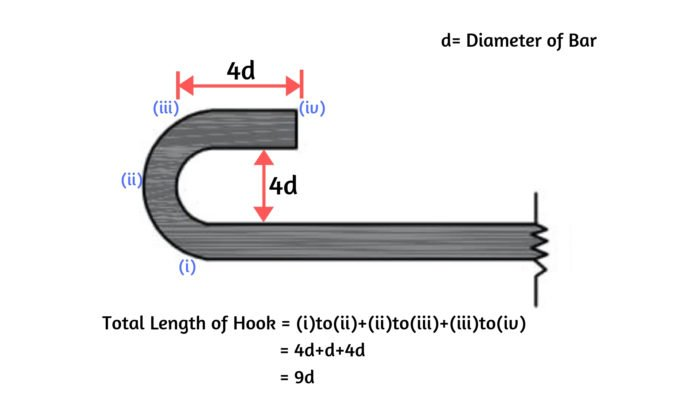From above fig, length of hook = [(Curved Portion) + 4d] = [(4d+d)+4d] = 9d

#### Example Calculation Considering Stirrup with the hooks at ends

For clear understanding, look at the below image for calculation of the total length of stirrup the with two hooks at ends.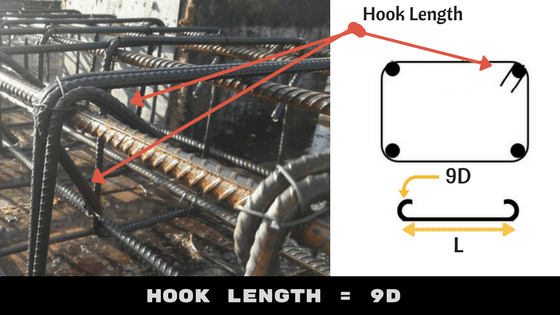Total Cutting Length of stirrup or tie = Total length of Bar + 2 x Hook Length (Two hooks)

Total Cutting Length = L+2(9d)

Therefore Total Cutting  length = L+18d (d is the Diameter of a bar)

Hope, now you are clear with the Hook length calculation.

## 2. Bend Length

The Bend length calculation is different for Cranked bars (bent up bars) and bends at corners.

The bars are usually cranked in Slabs and bars are bent at corners in Stirrups or ties.

### (i) Bend Length calculation in Cranked Bars

As  Shear stress is maximum at supports in Slab. To resist these stresses we usually crank the bars at the ends of supports in the slab. The below figure depicts the bent up bar in Slab. To calculate the bend length the below procedure is followed.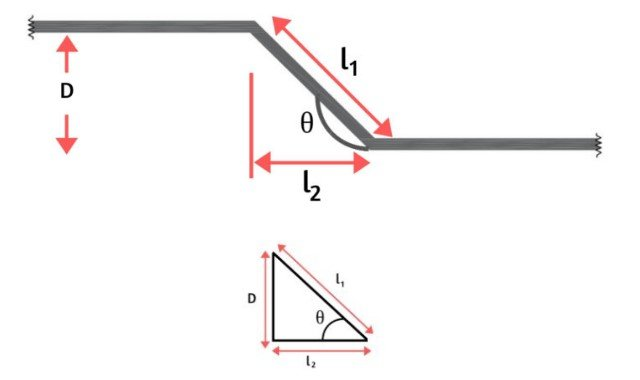From the above figure as the bar is bent at an angle θ0 the additional length (la) is introduced.

Where, la = l1 – l2–(i)

Tanθ = D/l2 ; Sinθ  = D/l1

Hence  l1  = D/Sinθ and l2 = D/tanθ

Therefore from (i)  :- la = D/Sinθ – D/tanθ

Giving different θ values as 300 , 450, 600  results different additional length  la values as below.

### la =D/Sinθ – D/tanθ

#### 2.42D

The additional length is added to the total length of the bar if the bars are cranked at a certain angle.

#### Example Calculation considering Bent up bar in Slab (Cranked bar)

To keep the crank bar in position, an extra bar of length (L/4) is provided below the crank bar as shown in the below figure.

2. ### Cutting length Determination Of a Spiral Or Helix Bar

Therefore, the total length of bar = L+0.42D+0.42D+(L/4)+(L/4) = 1.5L+0.84D

#### Remember D = Depth of Slab-Top Cover-Bottom cover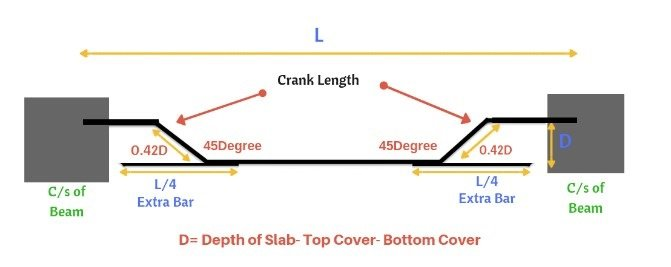### (ii) Bend Length calculation when bars are bent at corners

The important standards used while calculating the bend length at corners

1. 45° Bend length = 1d
2. 90° Bend length = 2d
3. 135° Bend length = 3d

Here, ‘d’ = Diameter of bar

### How to Calculate Staircase Concrete & Bar Bending Schedule And Staircase Reinforcement Details

#### Example Calculation considering stirrup with the bends at corners: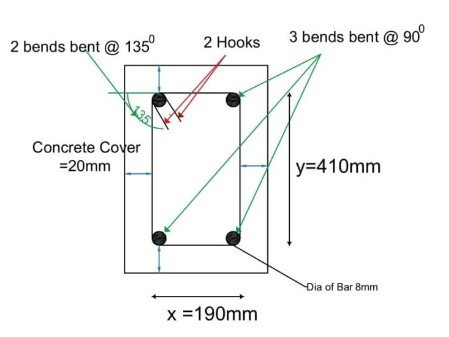From above fig, There are 3 bends which are bent at an angle of 900 and two bends are bent at an angle of 1350
Total bend length = 3 x 900 Bend length + 2 x 1350 Bend length = 3 x 2d + 2 x 3d = 12d = 12 x 8 = 96mm

Below table represents the total length of bar calculation for different types of bar shapes.
(If you are viewing the below table through mobile, scroll horizontally for a clear view)

#### Bent Up at one End only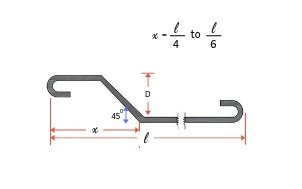#### Double Bent up Bar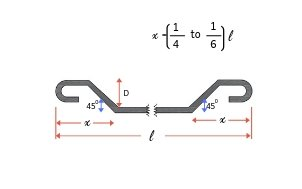## 3. Overlap Length / Lap Length in Reinforcement

The standard length of the Rebar is 12m. Suppose the height of the column is 20 m. To purvey this requirement, two bars of length 12m and 8m are overlapped (joined) with overlap length.

### How To Calculate The weight of Steel With Formula

Overlap Length for compression members (columns) = 50d

The Overlap Length for tension members (beams) = 40d

( d is the Diameter of the bar )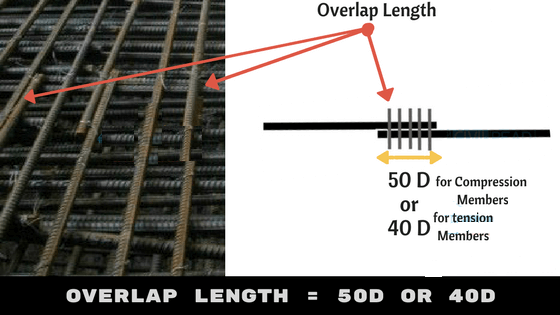Have You seen the below picture on your top floor of the buildings ? We generally project some length of Bar on the last floor i.e., 50D. It is the used for further constructions purpose. (Constructing a new floor)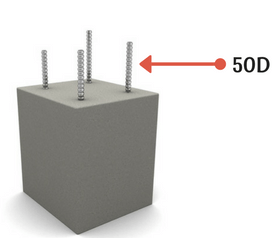# How to Prepare B.B.S

To understand clear, Here I am considering the below structural member of R.C.C Column and preparing a B.B.S for it.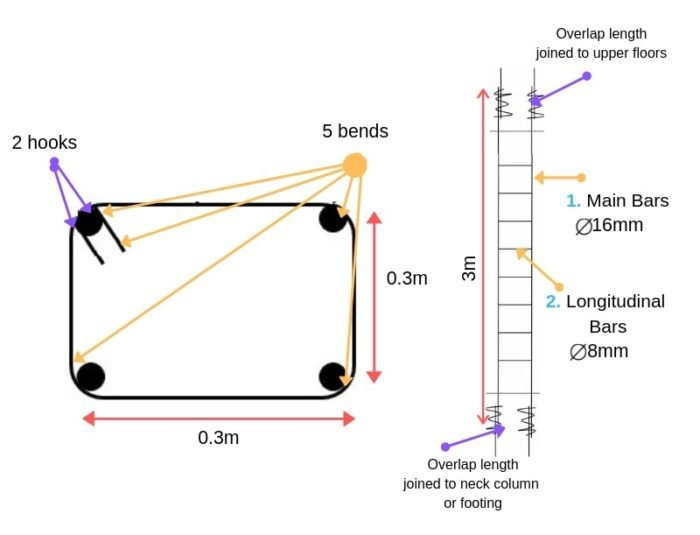### No. of Bars calculation:

Main bars = 4
To calculate the No. of longitudinal bars adopt spacing between bars is 0.1m
No. of Longitudinal bars = Length of column / Spacing = 3 / 0.1 = 30bars
Longitudinal bars = 30

### Cutting length calculation Main bars = 3m + 50d + 50d = 3 + 50×0.016 + 50×0.016 = 4.6m Stirrups: Hook length = 9d + 9d = 18d = 18×0.08=1.44m Bend length =3 x 900 Bend length + 2 x 1350 Bend length = 3 x 2d + 2 x 3d = 12d = 12 x 8 =0.096m

= l +hook length + bend length = 0.3+0.3+0.3+0.3 +0.144+0.096=1.44m

Hence for Main Bars = 4.6m ; Longitudinal bars = 1.44m

### Weight of steel bar for 1m = 1m x D2/162 Total weight of Main bars = 18.4 x 162/162 = 29Kgs Total weight of longitudinal bars = 43.2 x 82/162 =17Kgs

Total weight of steel bar required to do BBS of above column = 46Kgs

# Important Rules while preparing B.B.S

1. The bars used in building should be grouped together for each structural unit and listed separately for the each floor.
2. Bars are listed in numerical order.
3. To identify the bar in the bundle of the bars, each bar is uniquely labelled with reference details (Length of the bar, size of the bar, Shape of the bar)
4. The type of bar and shape of the bar should be in accordance with B8666.
5. It is essential that the bar mark reference on the label attached to a bundles of bars refers uniquely to a particular group or set of bars of defined length, size, shape and type used on the job.
6. The cutting length and bending length calculations are separately calculated and not included in the detailed lists. Like I have listed the Bar Bending details in a table & calculations are done separately.

# Use of B.B.S

1. B.B.S helps to estimate the total quantity of steel required for the construction of building or structure. It helps to quote for tender the cost incurred by steel.
2. Finding the cutting length and bending length in reinforcement detailing improves the quality of construction and minimize the wastage of steel, makes an economic construction
3. With the help of reinforcement drawings, cutting and bending can be done at the factory and transported to the site. This increases faster construction and reduces the total construction cost.
4. For site engineers, It becomes easy to verify the cutting length and bending length of the reinforcement before placing the concrete.

## What is The Advantages of Bar Bending Schedule ( B. B. S )

1 – When B.B.S is available, cutting and bending of reinforcement can be done at the factory & transported to the site. This increases faster executions at site & reduces construction time and cost due to less requirement of workers for bar bending. Bar bending also avoids the wastage of steel reinforcement (5 to 10%) & thus saves project costs.

2 – Using a B.B.S when used for Fe500, saves 10% more steels reinforcement compared to fe415.

3 – It improves the quality control at site as reinforcement is provided as per B.B.S which is prepared using the provisions of respective detailing standard codes.

### Calculation Of Staircase Concrete And Bar Bending Schedule (BBS) | Staircase Reinforcement Details

4 – It provides a better estimations of reinforcement steel requirement for each & every structural member which can be used to compute overall reinforcement requirement for entire project.

5 – It provides better stock management for reinforcement. The Steel requirement for the next phase of construction can be estimated with accuracy & procurement can be done. This prevents stocking of extra steel reinforcement at the site for a longer time, preventing corrosion of reinforcement in the case of coastal areas. It also the prevents a shortage of reinforcement for ongoing work by accurate estimation & thus concrete construction works can proceed smoothly.

6 – B.B.S is very much useful during auditing of reinforcement and provides checks on theft and pilferage.

7 – B.B.S can be used for reinforcement cutting, bending, and making a skeleton of a structural member before it can be placed at required position. Other activities such as excavations , P.C.C, etc.. can proceed parallel with this activities. So, overall project activities management becomes easy and reduces the time of construction. It becomes helpful in the preventing any damages due to construction time overrun.

### Quantity Calculation Of Round Staircase

8 – It provides benchmarks for the quantity & quality requirements for reinforcement & concrete works.

9 – B.B.S provides the steel quantity requirement much accurately and thus provides an option to optimize the design in case of cost overrun.

10 – It becomes easy for site engineers to verify and approve the bar bending and cutting length during inspection before placement of concrete with the use of B.B.S and helps in better quality control.

11 – It enables easy and fast preparation of bills of the construction works for clients and contractors.

12 –  The quantity of reinforcement to be used is calculated using engineering formulas and standard codes, so there is no option for approximate estimation of steel reinforcement.

### How to Calculate Staircase Concrete & Bar Bending Schedule And Staircase Reinforcement Details

13 – With the use of B.B.S, mechanization of cutting and bending of reinforcement can be done, again reducing the cost & time of project and dependency on the skilled labor requirement. It also improves the reliability of the accuracy of bar cutting and bending.

14 – When mechanized bar cutting & bending is used, the cost of reinforced concrete work per unit reduces and helps in the cost optimization of construction projects.

## Other Post

### THANKS.